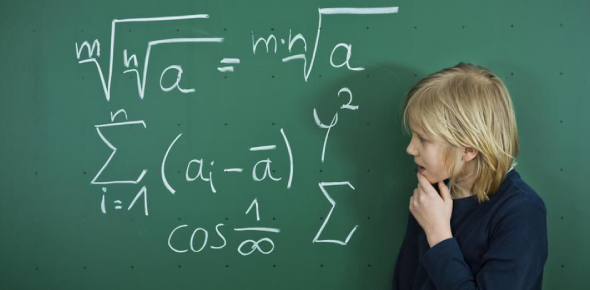# How Well Do You Know Your Math?

8 Questions | Total Attempts: 3761SettingsCreate your own QuizAll mathematics problems have a procedure for getting the correct solution. One way to ensure you are doing the right thing you should understand the rules, seek help and attempt each question. Do you have good mathematical skills? Why don’t you take up the quiz below and get to see how good you are? All the best and keep testing your skills!

• 1.
_____________ is the answer to an addition problem:
• A.

Sum

• B.

Difference

• C.

Product

• D.

Quotient

• 2.
Difference is the:
• A.

Answer to a multiplication problem

• B.

Answer to a division problem

• C.

Answer to a subtraction problem

• D.

• E.

I have no idea

• 3.
Before adding 1/3 and 2/9 I need to find a....
• A.

Mixed number

• B.

What 1 + 2 is

• C.

• D.

Common denominator

• 4.
When I add -9 and 7 together my answer is going to be
• A.

Positive

• B.

Negative

• C.

Over 100

• D.

Zero

• 5.
When multiplying two negative numbers my answer will ALWAYS be:
• A.

Negative

• B.

Zero

• C.

Positive

• D.

A decimal

• 6.
When I multiply 1 negative and 1 positive number together, my answer will ALWAYS be:
• A.

Zero

• B.

A decimal

• C.

Negative

• D.

Positive

• 7.
983.72105The 0 is in what place?
• A.

Ones

• B.

Tenths

• C.

Ten-thousandths

• D.

Hundredths

• E.

Thousandths

• 8.
Pick the correct symbol:576.889          576.800
• A.

• B.

>

• C.

=

Related TopicsBack to top
×

Wait!
Here's an interesting quiz for you.